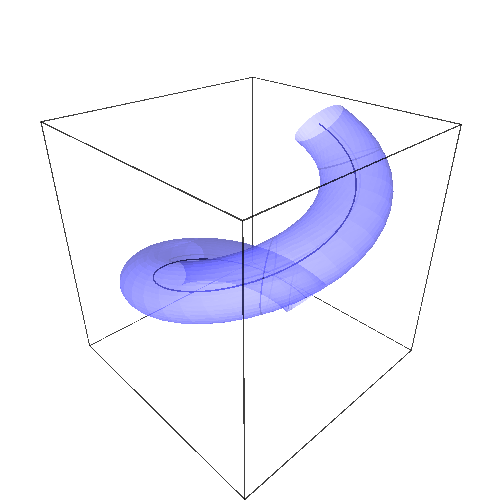# Tubular surface around curve

Hello,

I need help with drawing tubular surface around curve. It is for my college project but we never used program like Sage so I don't know how to use it properly. We have Curve c(t)=(2cos(t),2sin(t),0.5*t) and I need to draw tubular surface around it. I need to calculate frenet trihedron and use it in some formula for tubular surface. Formula is on this link: imgur.com/CUCu0da. My question is how do I make 3d projection out of this formula?

edit retag close merge delete

It is more like project because I get many points from this.

Okay. What do you call a "tubular surface around curve" ?

In plain English...

you should answer Emmanuel @Leon1403, because if it is just an appearance of a surface (a kind of plain tube surrounding the curve), it is easy , but on the other hand if it is a real surface, then me, personally I do not know how to do it !, but people on this site surely will know how to help you

Sorry, didn't see message sooner. English is not my native language but I will try to explain. This is how tube should look like. Curve should be inside of this tube. It is similar to charging cable. Plastic tube is surrounding wire.

Sort by » oldest newest most voted

Not (yet) a full answer (but see edit below if you are really stuck), but a (high-school level) hint, which, combined with FredericCs big fat one, should give you a solution :

Define the curve as a parametric callable symbolic expression :

t  = SR.var("t")
C(t) = vector([cos(t), sin(t), t/2])


Tangeant :

T(t) = vector(map(lambda u:u.diff(t), C(t)))
# Its norm is time independant...
Tn = T(t).norm().simplify_full()


Normal (= acceleration)

N(t) = vector(map(lambda u:u.function(t).diff(t,2), C(t)))
# Again, time-independent norm :
Nn = N(t).norm().simplify_full()


Binormal :

B(t) = T(t).cross_product(N(t))
# Ditto...
Bn = B(t).norm().simplify_full()


A vector of points where we want to plot Frenet's trihedron

tPts = srange(-pi, 11*pi/10, pi/5)


3D plot of the curve itself :

P1 = parametric_plot3d(C, (-pi, pi), color="black")


Plot the (normed) tangeants :

P2 = sum(map(lambda u:line((C(u),C(u)+T(u)/Tn), color="blue", arrow_head=True),
tPts))


The normals :

P3 = sum(map(lambda u:line((C(u),C(u)+N(u)/Nn), color="red", arrow_head=True),
tPts))


The binormals :

P4 = sum(map(lambda u:line((C(u),C(u)+B(u)/Bn), color="green", arrow_head=True),
tPts))


Now, perusing parametric_plot3d? will show you that this function can plot surfaces having two parameters. This should be more than enough for your goal...

EDIT : Do not read this before doing an honest attempt at finding the solution yourself ; otherwise, you'd lose the benefit of the homework).

This result can be used to get the desired plot. Let's define a description of the normal section of the tube (here a circle of radius r) : the vector (N(t)/Nn, B(t)/Bn) is an orthonormal basis of the plane normal to C(t). Therefore, this description is :

# Radius of a circular-section tube
var("r")
# Parametric desription of the normal section of the tube
Ts(t,theta)=N(t)/Nn*r*cos(theta)+B(t)/Bn*r*sin(theta)


and the description of any point of the tube is :

# A point of the tube
Tu(t,theta)=C(t)+Ts(t, theta)


which can be plotted (along with its center curve) by :

P1 + parametric_plot3d(Tu.subs(r==1/3), (-pi, pi), (0, 2*pi), opacity=0.5)


which should give you something along the lines of :Note that Sage's abilities in differential geometry may give you some radically different solutions. Left as an exercise for the (advanced) reader.

Bonus points : extrapolate to this... ;-)

HTH,

more

For the computation of the Frenet-Serret frame along a curve with Sage's differential geometry tools, see the section "Curvature and torsion of a curve in a Riemannian manifold" of https://doc.sagemath.org/html/en/refe...